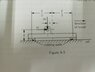# Find the distance x so that the two frictional forces are equal

Ithilrandir
Homework Statement:
A rocket-powered high speed sled running on rails employs pivot shoes between the sled and the rails, as shown in the figure. Each shoe has replaceable running pads at the heel and toe. The coefficient of friction between the running pads and the rail is μ. The rate at which the running pad material is worn off during the operation of the rocket sled is proportional to the friction force acting on the rubbing pad. If the shoe pivot point is at a given height h above the rail surface, at what horizontal distance, x, from the vertical centreline between the two rubbing pads should the pivot point P be placed do that the two rubbing pads will wear away at the same rate?

W: that portion of the weight of the rocket sled carried by the shoe in the diagram
H: horizontal component of force at the shoe pivot point
ℓ : total length between centres of the two rubbing pads
Relevant Equations:
...
Letting the normal force on the pad on the left be 1 and the one on the right be 2,

Normal force R1 + R2 = W

Since the sled is not rotating, net moment =0,

W(ℓ/2 + x) - Hh - R1ℓ =0

R1 = W/2 + Wx/ℓ - Hh/ℓ

Since the rate of the pads being worn is proportional to the frictional force, for the rate to be the same the frictional force on both sides needs to be the same as well.

R1 = R2 = W/2

Putting this back in the previous equation,

You get Wx/ℓ = Hh/ℓ,
x = Hh/W

However in the answer key the answer is μh. What am I missing in my steps?

#### Attachments

•IMG_20210216_144917.jpg
29 KB · Views: 40

Homework Helper
Gold Member
2022 Award
It is not a statics question. There is a horizontal acceleration.
If you take moments about a point not on the same horizontal as the mass centre, there is a net moment producing an angular acceleration about the point.

Ithilrandir
It is not a statics question. There is a horizontal acceleration.
If you take moments about a point not on the same horizontal as the mass centre, there is a net moment producing an angular acceleration about the point.
Does this mean that if I take the pivot at the centre of mass, there would not be any net moment?

Ithilrandir
Does this mean that if I take the pivot at the centre of mass, there would not be any net moment?
I seemed to have gotten the answer by taking the pivot at the point P. The only thing that is off is that I got a negative answer.

Homework Helper
Gold Member
2022 Award
I seemed to have gotten the answer by taking the pivot at the point P. The only thing that is off is that I got a negative answer.
Well, that's progress.

•Delta2
Ithilrandir
Well, that's progress.

I noticed I had just been careless in my arithmetic and forgot to change the signs when bringing terms from one side to the next. So my answer has no issues.

Homework Helper
Gold Member
2022 Award
I noticed I had just been careless in my arithmetic and forgot to change the signs when bringing terms from one side to the next. So my answer has no issues.
Very good.
Do you understand about the angular acceleration?

Ithilrandir
Very good.
Do you understand about the angular acceleration?
I didn't make use of angular acceleration in my calculations, so no. I just took into account of friction when I calculated the moment about point P.

Homework Helper
Gold Member
2022 Award
I didn't make use of angular acceleration in my calculations, so no. I just took into account of friction when I calculated the moment about point P.
Sure, but I am asking if you understand why you need to allow for an angular acceleration if you choose some other axis.

Ithilrandir
Sure, but I am asking if you understand why you need to allow for an angular acceleration if you choose some other axis.
I'm thinking it's because the net movement is from the centre of mass, motions from other points of reference will essentially have an added rotational element.

•